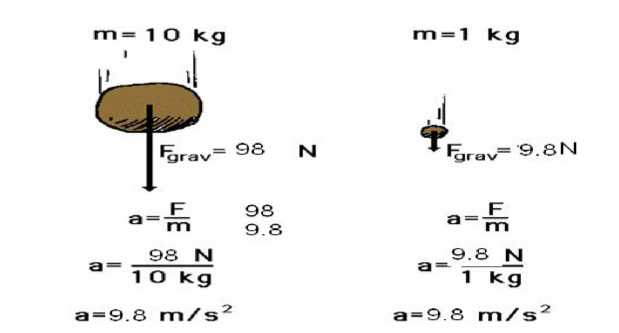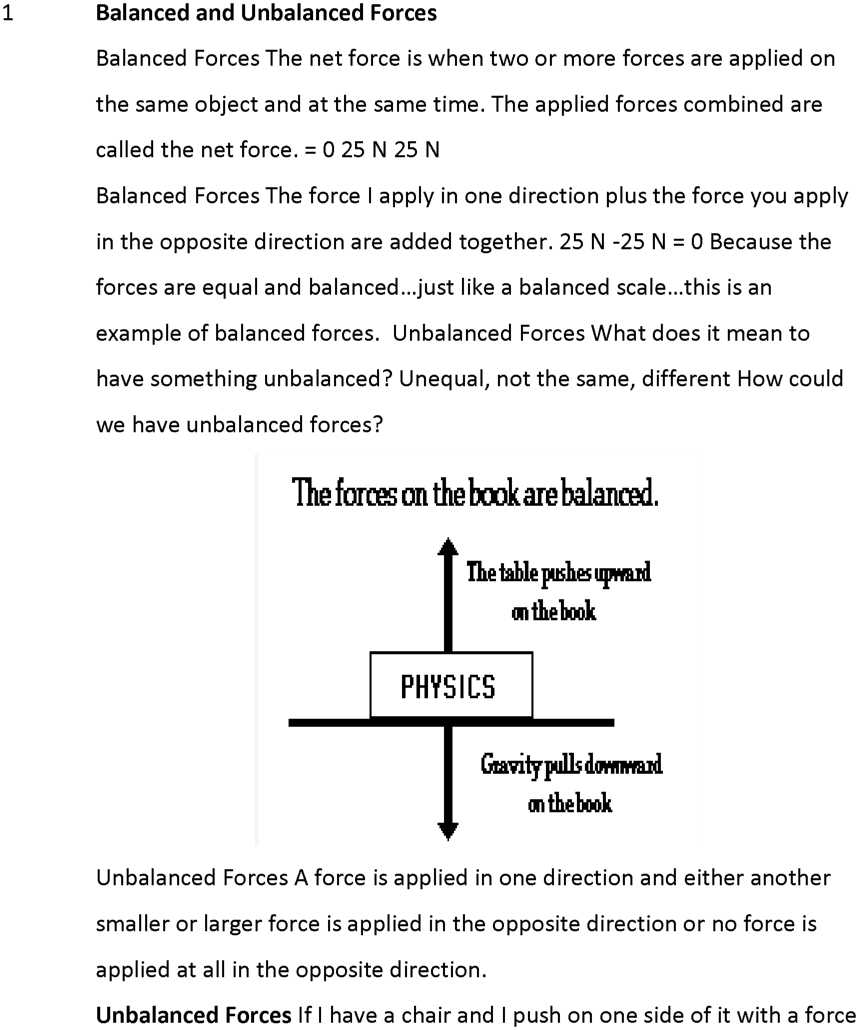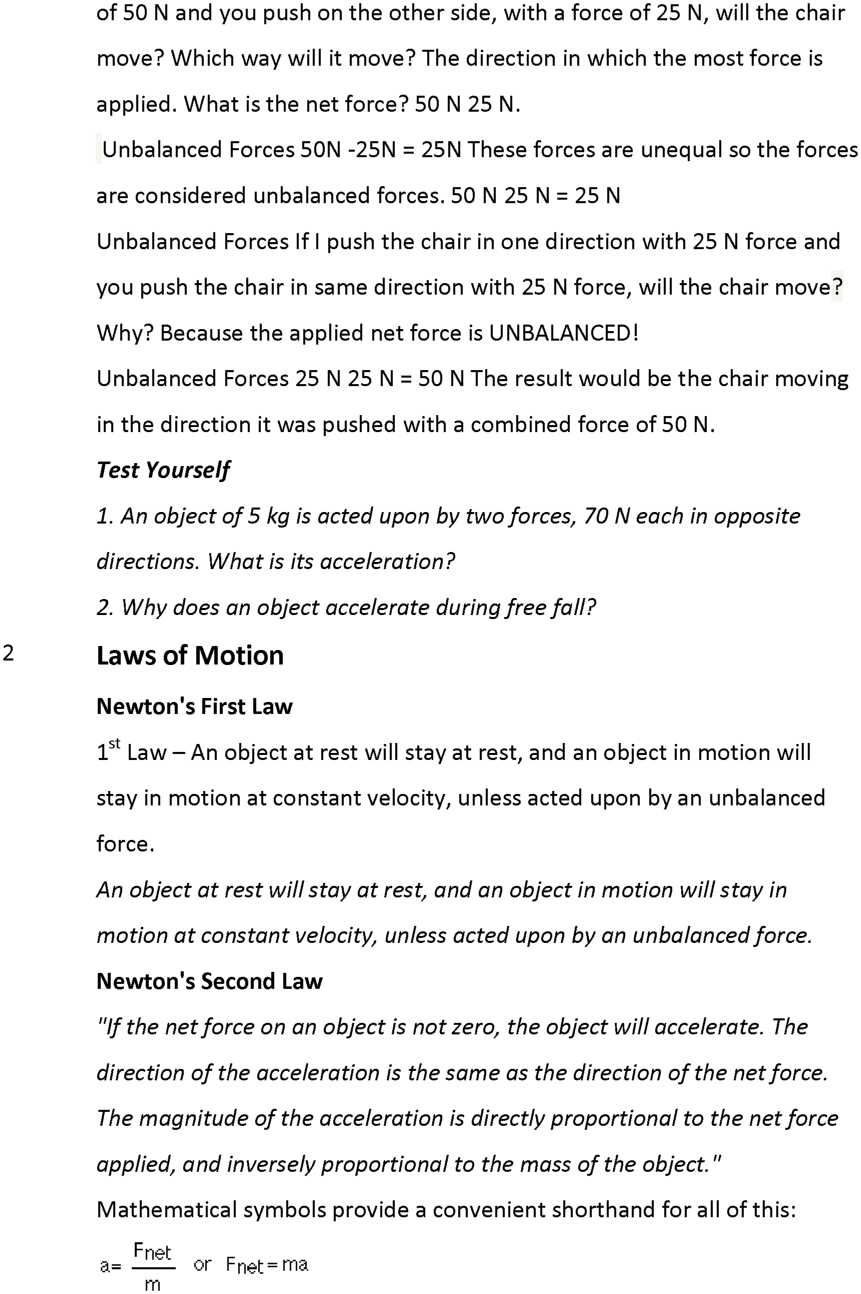# Force and Laws of Motion Notes Class 9th Science Chapter 9

0
2026CHAPTER -9 “Force & Laws Of Motion”

KEY CONCEPTS [ *rating as per the significance of concept]

 1 Balanced and Unbalanced Forces *** 2 Laws of Motion ***** 3 Inertia and Mass ***** 4 Conservation of Momentum ****The Effect of Mass

A force applied to an automobile will not have the same effect as the same force applied to a pencil. An automobile resists accelerating much more than a pencil does, because it has more inertia, or mass.

The acceleration of an object depends not only on how hard you push on it, but also on how much the object resists being pushed.

What is the effect of mass on acceleration? This, too, turns out to be quite simple (I wonder why…). For the same force, an object with twice the mass will have half the acceleration. If it had three times the mass, the same force will produce one-third the acceleration. Four times the mass gives one-fourth of the acceleration, and so on.

This type of relationship between quantities (double one, get half the other) is called an inverse proportion or inverse variation. In other words, then:

Newton’s Second Law of Motion The acceleration of an object is dependent upon both force and mass. Thus, if the colliding objects have unequal mass, they will have unequal accelerations as a result of the contact force which results during the collision.

Newton’s Third Law Newton’s Third Law is stated as:

For every action there is an equal and opposite reaction.

“action…reaction” means that forces always occur in pairs. (Forces are interactions between objects, like conversations are interactions between people.)

Single, isolated forces never happen. The two forces involved are called the “action force” and the “reaction force.”

These names are unfortunate for a couple of reasons :

Either force in an interaction can be the “action” force or the “reaction” force

The action and reaction forces exist at the same time.

“equal” means

Both forces are exactly the same size. They are equal in magnitude.

Both forces exist at exactly the same time. They both start at exactly the same instant, and they both stop at exactly the same instant. They are equal in time.

“opposite” means that the two forces always act in opposite directions – exactly 180o apart.

Newton’s third law of motion In every interaction, there is a pair of forces acting on the two interacting objects. The size of the force on the first object equals the size of the force on the second object. The direction of the force on the first object is opposite to the direction of the force on the second object. Forces always come in pairs – equal and opposite action- reaction force pairs.

Newton’s third law of motion applied to collisions between two objects. In a collision between two objects, both objects experience forces which are equal in magnitude and opposite in direction. Such forces cause one object to speed up (gain momentum) and the other object to slow down (lose momentum). According to Newton’s third law, the forces on the two objects are equal in magnitude.

Test Yourself

1. Can action reaction balance each other?
2. What does a force do?

3. Inertia and Mass

Inertia is the tendency of an object to resist any change in its motion. An object will continue to move at the same speed in the same direction

unless acted upon by an unbalanced force. Inertia & Mass Inertia & Mass of a bowling ball rolled down the road would eventually come to a stop. Friction is an unbalanced force that causes the ball to stop or slow down. Without friction, the ball would keep going.

Inertia & Mass of a bowling ball and a tennis ball have the same inertia. Inertia & Mass If you had a tennis racket and I threw tennis ball at you, what would happen? If you had a tennis racket and I threw a bowling ball at you, what would happen? Why could you change the motion of the tennis ball but not the motion of the bowling ball?

Mass is the amount of matter in an object. A bowling ball has more mass than a tennis ball. The greater the mass of an object the greater its inertia. Mass is the measurement of inertia.

Test Yourself

1. Why do we fall forward if we alight from a moving bus?
2. Why does an athlete run for some distance before long jump?
3. Conservation of Momentum

Law of Conservation of Momentum

In a closed system, the vector sum of the momenta before and after an impact must be equal.

Before After

m1v1 +m2v2 = m1v1‘ + m2v2

Internal and External Forces

QUESTION BANK

One Mark questions

1. Define momentum.
2. State first law of motion.
3. What is inertia?
4. Can action and reaction balance each other?
5. How does one climb up a rope?
6. Why cannot we walk in space?
7. What does rate of change of momentum represent?
8. Why do we continuously paddle to keep the cycle moving?
9. Why does a scooter tend to skid while executing a sharp turn?
10. Which one would have more inertia : 10 kg mass & 5 kg mass?

Two Marks questions

1. Explain the functioning of shockers in cars.
2. How much force is needed to pull an object of mass 40 kg in vertically upward direction with acceleration of 2.2 m / s2.
3. Why does a fan keep moving for sometime when switched off?
4. What do you mean by conservation of momentum?
5. Inflated balloon lying on the surface of a floor moves forward when pricked with a pin. Why?

Three Marks questions

1. An iron sphere of mass 10 kg is dropped from a height of 80 cm, if ‘g’ = 10 m / s2. Calculate the momentum transferred to the ground by the body.
2. What would be the force required to stop a car of mass 1000 kg and a loaded truck of mass 10,000 kg in 2 seconds each moving with velocity 5 m / s.
3. Deduce law of conservation of momentum using third law of motion.

Five Mark questions

1. Name and define three different types of inertia & give an example of each.

Previous articleMotion Notes Class 9th Science Chapter 8
Next articleGravitation Notes Class 9th Science Chapter 10